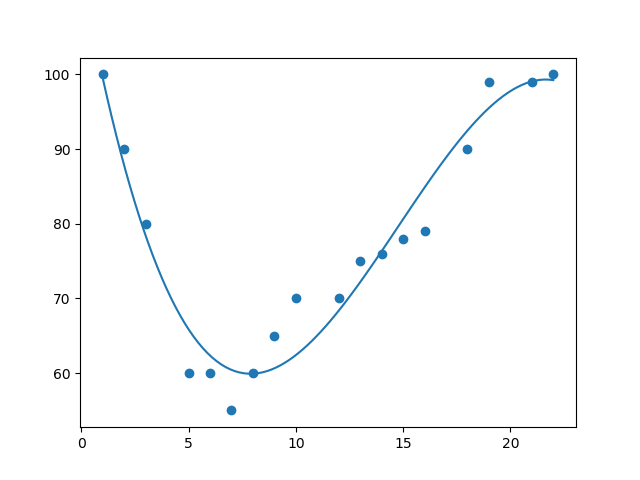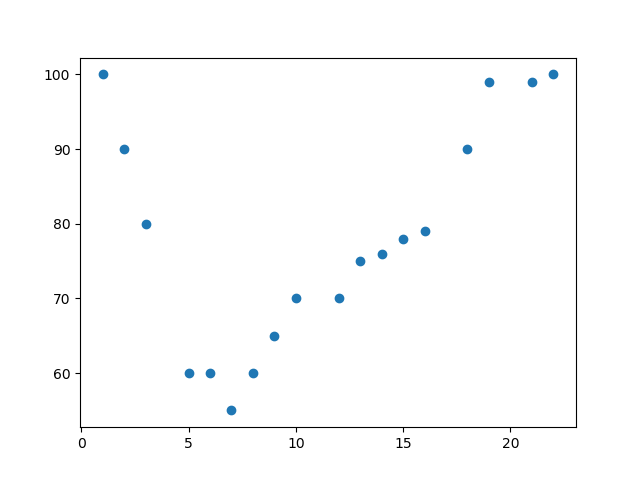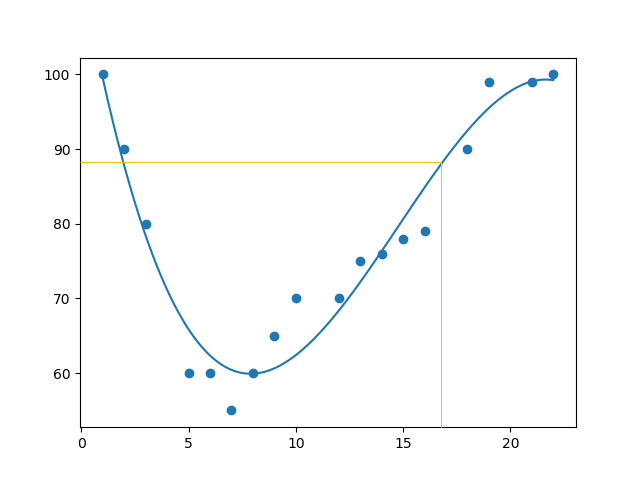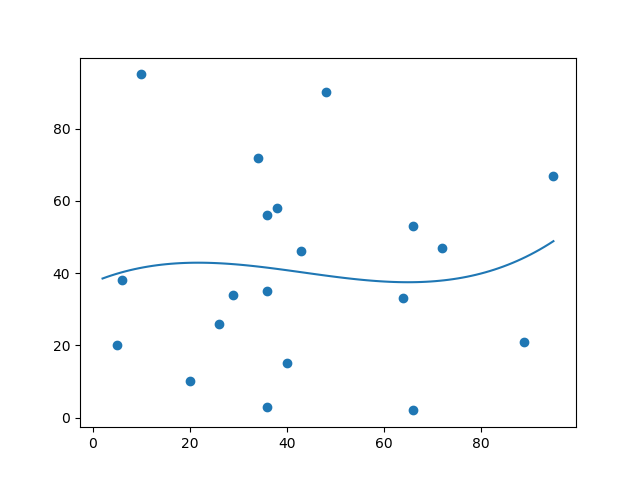## Python Tutorial

Python HOME Python Intro Python Get Started Python Syntax Python Comments Python Variables Python Data Types Python Numbers Python Casting Python Strings Python Booleans Python Operators Python Lists Python Tuples Python Sets Python Dictionaries Python If...Else Python While Loops Python For Loops Python Functions Python Lambda Python Arrays Python Classes/Objects Python Inheritance Python Iterators Python Polymorphism Python Scope Python Modules Python Dates Python Math Python JSON Python RegEx Python PIP Python Try...Except Python User Input Python String Formatting

## File Handling

Python File Handling Python Read Files Python Write/Create Files Python Delete Files

## Python Modules

NumPy Tutorial Pandas Tutorial SciPy Tutorial Django Tutorial

## Python Matplotlib

Matplotlib Intro Matplotlib Get Started Matplotlib Pyplot Matplotlib Plotting Matplotlib Markers Matplotlib Line Matplotlib Labels Matplotlib Grid Matplotlib Subplot Matplotlib Scatter Matplotlib Bars Matplotlib Histograms Matplotlib Pie Charts

## Machine Learning

Getting Started Mean Median Mode Standard Deviation Percentile Data Distribution Normal Data Distribution Scatter Plot Linear Regression Polynomial Regression Multiple Regression Scale Train/Test Decision Tree Confusion Matrix Hierarchical Clustering Logistic Regression Grid Search Categorical Data K-means Bootstrap Aggregation Cross Validation AUC - ROC Curve K-nearest neighbors

## Python MySQL

MySQL Get Started MySQL Create Database MySQL Create Table MySQL Insert MySQL Select MySQL Where MySQL Order By MySQL Delete MySQL Drop Table MySQL Update MySQL Limit MySQL Join

## Python MongoDB

MongoDB Get Started MongoDB Create Database MongoDB Create Collection MongoDB Insert MongoDB Find MongoDB Query MongoDB Sort MongoDB Delete MongoDB Drop Collection MongoDB Update MongoDB Limit

## Python Reference

Python Overview Python Built-in Functions Python String Methods Python List Methods Python Dictionary Methods Python Tuple Methods Python Set Methods Python File Methods Python Keywords Python Exceptions Python Glossary

## Module Reference

Random Module Requests Module Statistics Module Math Module cMath Module

## Python How To

Remove List Duplicates Reverse a String Add Two Numbers

## Python Examples

Python Examples Python Compiler Python Exercises Python Quiz Python Bootcamp Python Certificate

# Machine Learning - Polynomial Regression

## Polynomial Regression

If your data points clearly will not fit a linear regression (a straight line through all data points), it might be ideal for polynomial regression.

Polynomial regression, like linear regression, uses the relationship between the variables x and y to find the best way to draw a line through the data points.## How Does it Work?

Python has methods for finding a relationship between data-points and to draw a line of polynomial regression. We will show you how to use these methods instead of going through the mathematic formula.

In the example below, we have registered 18 cars as they were passing a certain tollbooth.

We have registered the car's speed, and the time of day (hour) the passing occurred.

The x-axis represents the hours of the day and the y-axis represents the speed:

### Example

Start by drawing a scatter plot:

import matplotlib.pyplot as plt

x = [1,2,3,5,6,7,8,9,10,12,13,14,15,16,18,19,21,22]
y = [100,90,80,60,60,55,60,65,70,70,75,76,78,79,90,99,99,100]

plt.scatter(x, y)
plt.show()

### Result:Run example »

### Example

Import `numpy` and ``` matplotlib``` then draw the line of Polynomial Regression:

import numpy
import matplotlib.pyplot as plt

x = [1,2,3,5,6,7,8,9,10,12,13,14,15,16,18,19,21,22]
y = [100,90,80,60,60,55,60,65,70,70,75,76,78,79,90,99,99,100]

mymodel = numpy.poly1d(numpy.polyfit(x, y, 3))

myline = numpy.linspace(1, 22, 100)

plt.scatter(x, y)
plt.plot(myline, mymodel(myline))
plt.show()

### Result:Run example »

### Example Explained

Import the modules you need.

You can learn about the NumPy module in our NumPy Tutorial.

You can learn about the SciPy module in our SciPy Tutorial.

`import numpyimport matplotlib.pyplot as plt`

Create the arrays that represent the values of the x and y axis:

``` x = [1,2,3,5,6,7,8,9,10,12,13,14,15,16,18,19,21,22]y = [100,90,80,60,60,55,60,65,70,70,75,76,78,79,90,99,99,100] ```

NumPy has a method that lets us make a polynomial model:

``` mymodel = numpy.poly1d(numpy.polyfit(x, y, 3))```

Then specify how the line will display, we start at position 1, and end at position 22:

``` myline = numpy.linspace(1, 22, 100)```

Draw the original scatter plot:

``` plt.scatter(x, y)```

Draw the line of polynomial regression:

``` plt.plot(myline, mymodel(myline))```

Display the diagram:

``` plt.show()```

## R-Squared

It is important to know how well the relationship between the values of the x- and y-axis is, if there are no relationship the polynomial regression can not be used to predict anything.

The relationship is measured with a value called the r-squared.

The r-squared value ranges from 0 to 1, where 0 means no relationship, and 1 means 100% related.

Python and the Sklearn module will compute this value for you, all you have to do is feed it with the x and y arrays:

### Example

How well does my data fit in a polynomial regression?

import numpy
from sklearn.metrics import r2_score

x = [1,2,3,5,6,7,8,9,10,12,13,14,15,16,18,19,21,22]
y = [100,90,80,60,60,55,60,65,70,70,75,76,78,79,90,99,99,100]

mymodel = numpy.poly1d(numpy.polyfit(x, y, 3))

print(r2_score(y, mymodel(x)))
Try if Yourself »

Note: The result 0.94 shows that there is a very good relationship, and we can use polynomial regression in future predictions.

## Predict Future Values

Now we can use the information we have gathered to predict future values.

Example: Let us try to predict the speed of a car that passes the tollbooth at around the time 17:00:

To do so, we need the same `mymodel` array from the example above:

``` mymodel = numpy.poly1d(numpy.polyfit(x, y, 3))```

### Example

Predict the speed of a car passing at 17:00:

import numpy
from sklearn.metrics import r2_score

x = [1,2,3,5,6,7,8,9,10,12,13,14,15,16,18,19,21,22]
y = [100,90,80,60,60,55,60,65,70,70,75,76,78,79,90,99,99,100]

mymodel = numpy.poly1d(numpy.polyfit(x, y, 3))

speed = mymodel(17)
print(speed)
Run example »

The example predicted a speed to be 88.87, which we also could read from the diagram:Let us create an example where polynomial regression would not be the best method to predict future values.

### Example

These values for the x- and y-axis should result in a very bad fit for polynomial regression:

import numpy
import matplotlib.pyplot as plt

x = [89,43,36,36,95,10,66,34,38,20,26,29,48,64,6,5,36,66,72,40]
y = [21,46,3,35,67,95,53,72,58,10,26,34,90,33,38,20,56,2,47,15]

mymodel = numpy.poly1d(numpy.polyfit(x, y, 3))

myline = numpy.linspace(2, 95, 100)

plt.scatter(x, y)
plt.plot(myline, mymodel(myline))
plt.show()

### Result:Run example »

And the r-squared value?

### Example

You should get a very low r-squared value.

import numpy
from sklearn.metrics import r2_score

x = [89,43,36,36,95,10,66,34,38,20,26,29,48,64,6,5,36,66,72,40]
y = [21,46,3,35,67,95,53,72,58,10,26,34,90,33,38,20,56,2,47,15]

mymodel = numpy.poly1d(numpy.polyfit(x, y, 3))

print(r2_score(y, mymodel(x)))
Try if Yourself »

The result: 0.00995 indicates a very bad relationship, and tells us that this data set is not suitable for polynomial regression.